# Band-pass Filter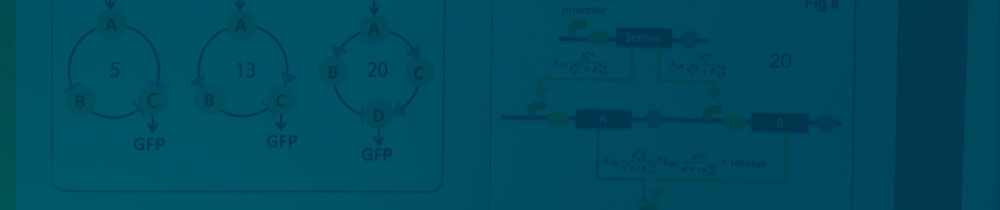# Band-pass Filter

Introduction

Here we demonstrate how we rationally designed the circuit network and determined appropriate protein regulator candidates to construct our Band-pass Filter. First we selected three circuit networks possessing an incoherent feed-forward loop as their core topology and constructed Ordinary Differential Equations (ODEs) describing their kinetic behavior. Based on the steady state solutions of these equations, we further evaluated the robustness of these circuit networks by calculating their Q values using randomly sampled parameter sets. We then chose the network with an outstanding Q value and analyzed its parameter preference through a parameter sensitivity analysis. Equipped with such knowledge, we were able to pick out regulatory proteins with kinetic/dynamic parameters close to the chosen network's preferred values. With all these efforts, we finally determined the genetic circuit for our Band-pass Filter.

Selecting Network Topologies

There are a lot of potential Band-pass Filter circuit networks with an incoherent feed-forward loop (for specific definition, view Project: Band-pass Filter ) as its core topology. The simplest one is a two-node network consisting of a positive loop and a negative loop (Fig. 1a). But it’s too difficult to make a transcriptional factor both activator and repressor at the same time. So we discarded such a network despite it simplicity.

So we set out to look for topologies with three or more nodes based on two criteria: first, considering functioning mechanisms of real protein regulators, the input node must regulate other nodes in a uniform manner, either all inhibiting or all promoting; second, to lessen the manual work required to construct the circuit, we require both the positive and negative feed-forward loop to contain no more than one internode. In the end, we decided to analyze three topologies in detail, two of them have three nodes and one has four nodes (Fig. 1b, 1c, 1d).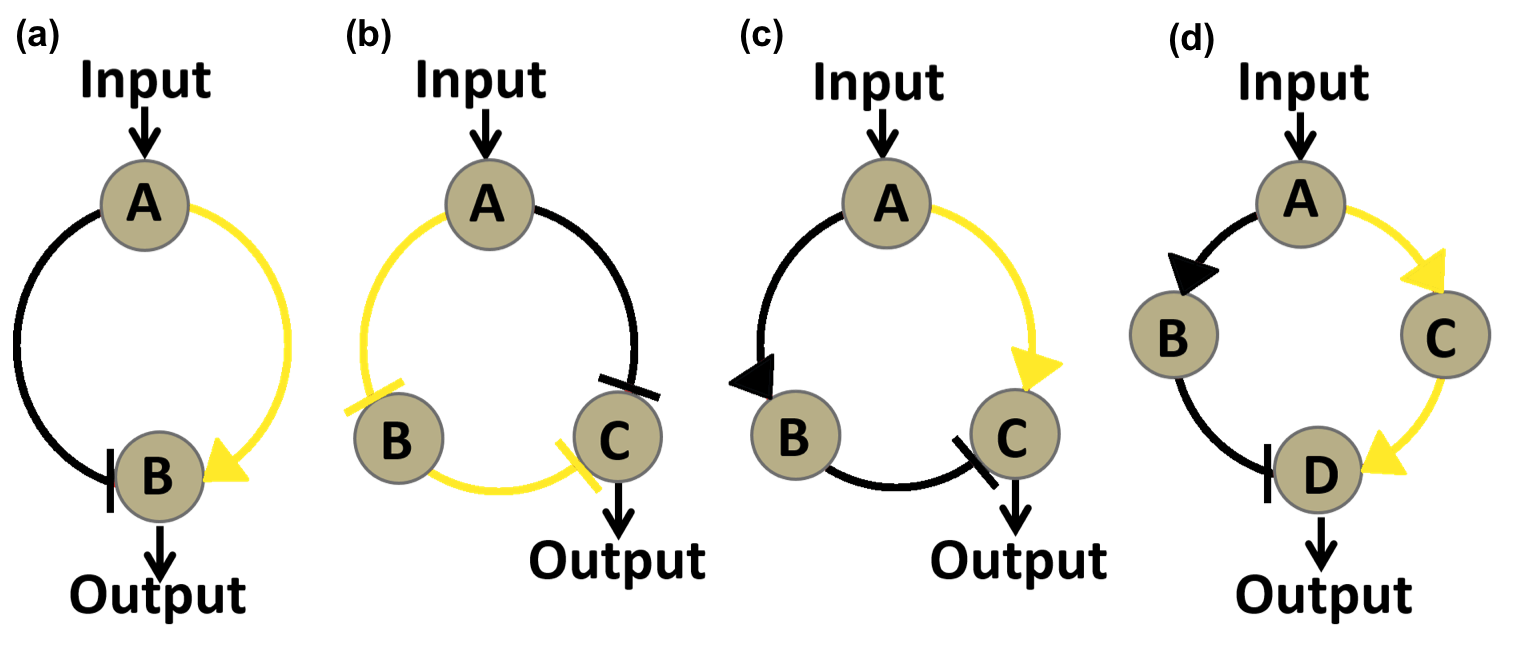Figure. 1. Graphs of four circuit networks we considered in our modeling. Each node represent a regulatory protein, either an activator or and repressor. All four networks possess incoherent feed-forward loops as core topology. Components of the activating half of an incoherent feed-forward loop are marked as green while components of repressing half are marked as black. a. The simplest two node circuit possessing an incoherent feed-forward loop. In this network, Input node A functions both as an activator and an repressor, so it has to be eliminated. b, c, Three-node networks taken into consideration. The principal difference between these two networks is the regulatory function of input node A. b, Three-node network where input node A functions as repressor. A directly repress output node C while indirectly activating it by inhibiting B, which represses C directly. c, Three-node network where input node A functions as activator. A directly repress output node C while indirectly activating it by activating B, which represses C directly. d, Four-node network taken into consideration. A indirectly activates output node D by activating C which activates D, while indirectly represses output by activating B that inhibits D.

ODE Analysis of Circuit Networks

After determining three typical circuit networks that might function as Band-pass Filters, we used Ordinary Differential Equations (ODEs) to analyze the three topology and their parameter preferences.

Our ODE model of the circuit networks was established based on the following assumptions:

1. Only transcription and translation process is taken into consideration. The equations only contain accumulation and degradation of the proteins represented by the nodes.
2. The interactions between nodes are described using Hill functions. We multiplied the Hill functions describing the individual regulating effects of activator and repressor in order to simulate the synergic regulating effect of the two transcription factors when bound to the same promoter.
3. The protein represented by the input node is constitutively expressed at a fixed level.
4. Leakage is present only in the expression of reporter gene (output node).

The ODE equations we used to simulate the three circuit networks are listed as following:

Circuit Network 1:Note: Since A is expressed at a fixed level, its influence on the network can be absorbed into other constants in the model, so it doesn't appear in the equations written above.

Letting the differential term on the left handed side equal to zero, the steady state solution of the equations can be solved as following:Circuit Network 2: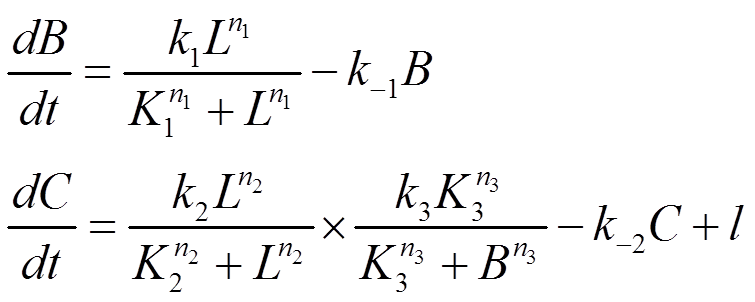Note: For reason same as that described in Circuit Network 1, expression level of A doesn't appear in the equations written above.

The steady state solution of the equations can be solved as following: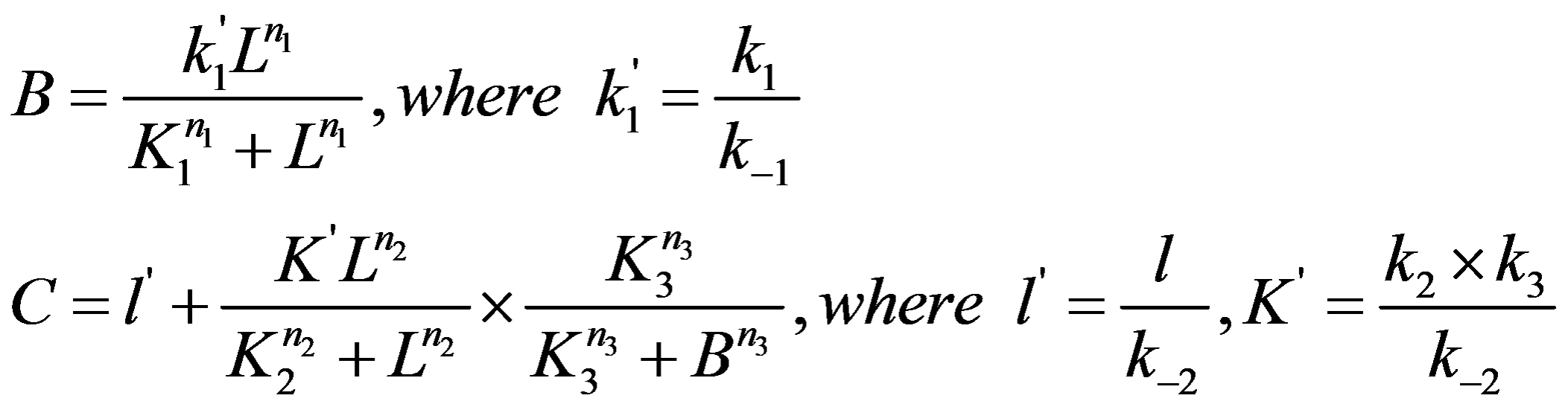Circuit Network 3:Note: For reason same as that described in Circuit Network 1, expression level of A doesn't appear in the equations written above.

The steady state solution of the equations can be solved as following: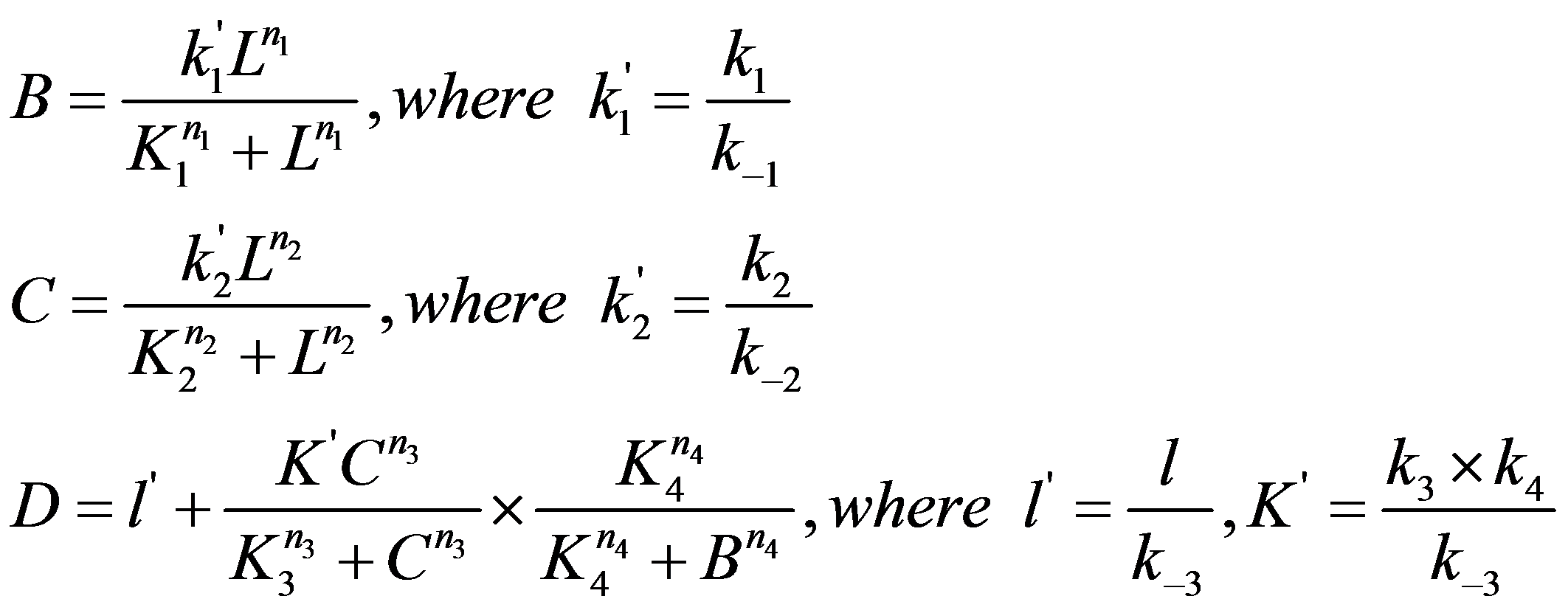In the equations written above, k in lowercase are used to denote maximum expression rate constant of individual regulation loops. K in uppercase are used to denote the transition point of different Hill functions. A, B, C, D are used to denote expression level of proteins represented by corresponding nodes. n in lowercase denote Hill coefficient of different Hill functions. l in lowercase denote leakage terms. L in uppercase denote input ligand concentration.
The next step is to use ODE equations given above to find out the most robust circuit network. In order to do this, we applied 10,000 sets of randomly sampled network parameters to each of the three topologies and evaluated their behavior according to two important features: induction fold and peak width (Fig. 2). Parameter sets resulting in a high induction fold (greater than 32) and a reasonable peak width (less than 4 and greater than 0.5) are recorded separately for each topology.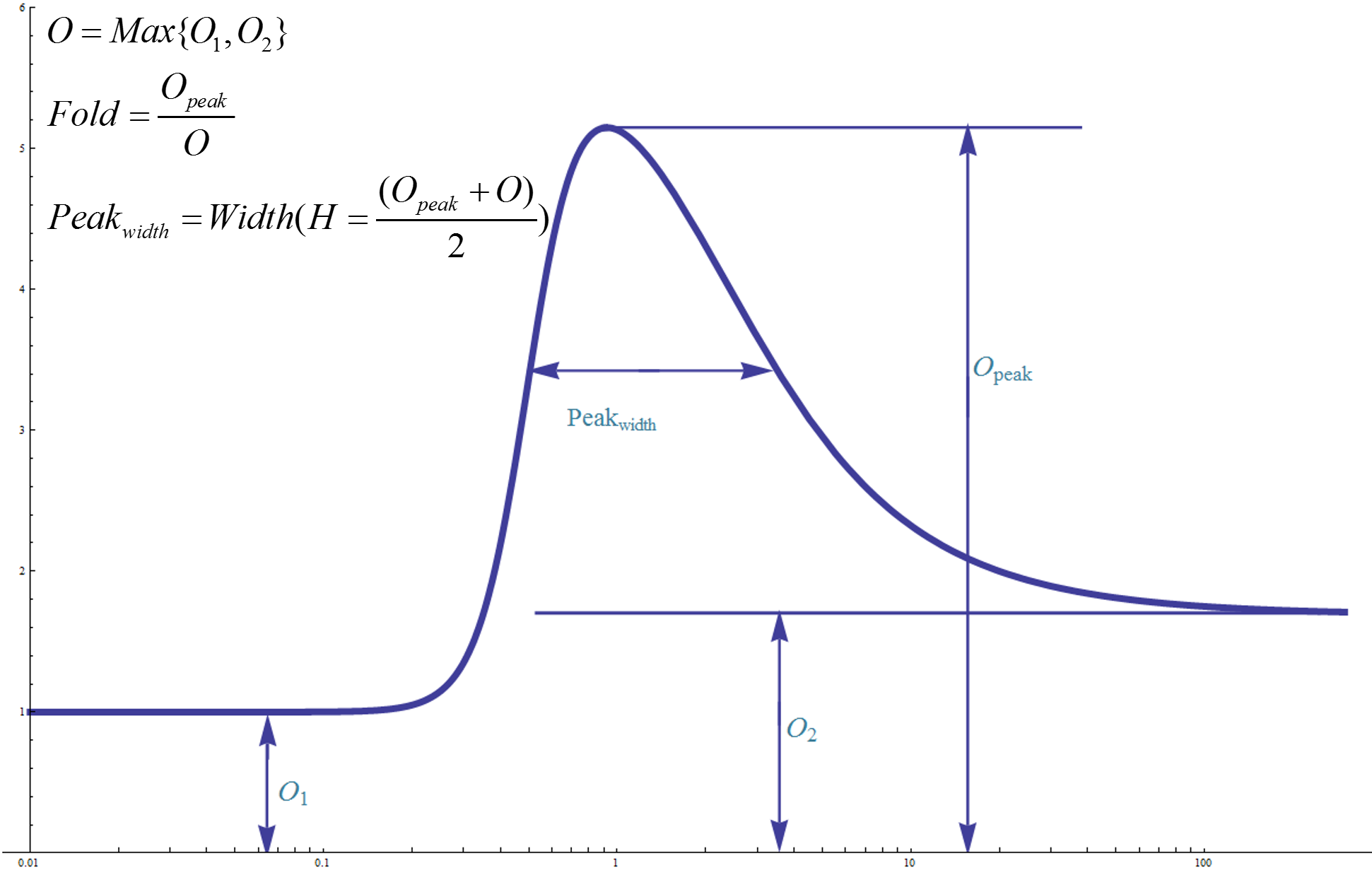Figure. 2. Graph illustration of the definition of induction fold and peak width. Left basal value O1 equals to y-axis intercept of the dose-response curve's horizontal asymptotic line as input intensity tends to zero. Right basal value O2 equals to y-axis intercept of the dose-response curve's horizontal asymptotic line as input intensity tends to infinity. Effective basal value O is defined as the greater one among O1 and O2. Opeak equals to peak value of the dose-response curve. Induction ration equals to Opeak divided by O. Peak width is defined as the distance between the two points with y coordinate equal to (Opeak+O)/2. Induction fold and peak width is the two most important judging criteria used in our modeling.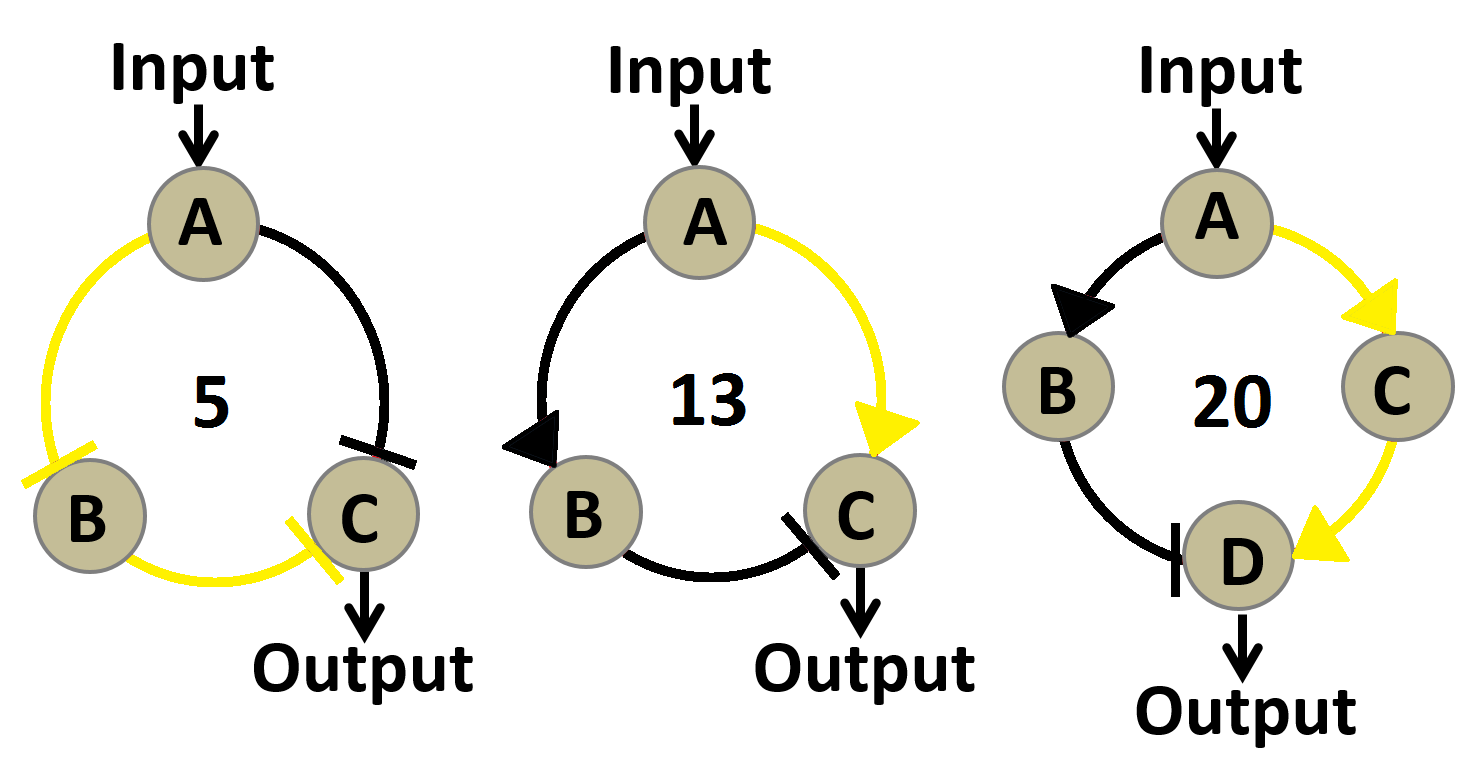Figure. 3.Graphs of three circuit networks we evaluated in our ODE based robustness analysis. The number of parameter sets capable of generating desirable induction fold(greater than 32) and peak width (less than 4 and greater than 0.5) are written in the middle of each network graph. A larger number of effective parameter sets indicates a more robust circuit network.

Results of robustness analysis are shown in Figure 2. 20 out 10000 sets of parameters applied to circuit network 3 generated a desirable performance. The number of effective parameter sets for circuit network 1 and 2 are 5 and 13 respectively. This indicates that circuit network 3 may function in a larger proportion of the parameter space, meaning it would be more likely than the other two to work well in noisy biological context and might require less effort on fine-tuning individual components of the circuit network. So we chose the third circuit network as the blueprint for our Band-pass Filter.

Parameter Sensitivity Analysis

Having decided which circuit network to use, our next move is to determine which proteins to choose for individual nodes in the circuit network to construct a Band-pass Filter. First we decided to use parameter sensitivity analysis to figure out which parameters in the circuit network are most sensitive to perturbation. These parameters are crucial for own design because they must be carefully controlled within a narrow range to make sure our circuit would function properly. Once we have figured out all sensitive parameters, we may facilitate our design by choosing proteins with desirable sensitive parameter value.
First we drew the blueprint for our Band-pass Filter genetic circuit design. For our application purpose, the input node was supposed to represent transcription regulators that are responsive to environmental aromatic compounds. We also fixed sfGFP reporter as the output node. We mainly focused on determining proper candidates for node A and B through sensitive parameter analysis.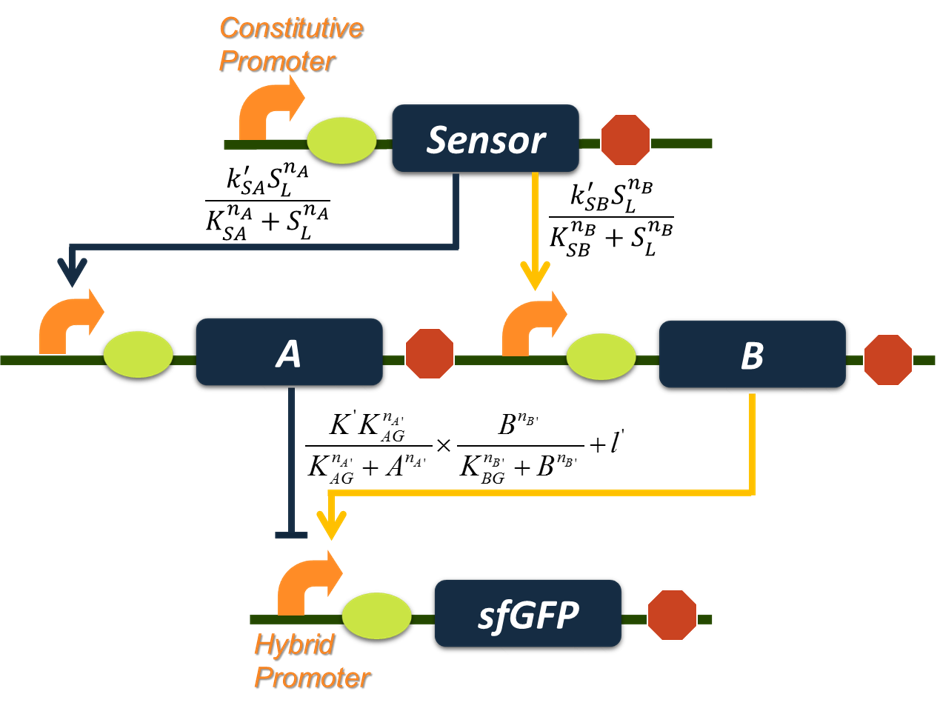Figure. 4. Blueprint for the construction of Band-pass Filter circuit and a graph summary of equations we use for stimulation. The sensor for input node is supposed to be a transcription regulator capable of responding to aromatic compounds, and we fixed sfGFP reporter as the output node; protein candidates for repressor node A and activator node B remain to be determined based on information gathered from parameter sensitivity analysis.

In order to be more specific in our parameter sensitivity analysis, we re-wrote the ODEs for the Band-pass Filter as following:And steady state solution can be solved as following: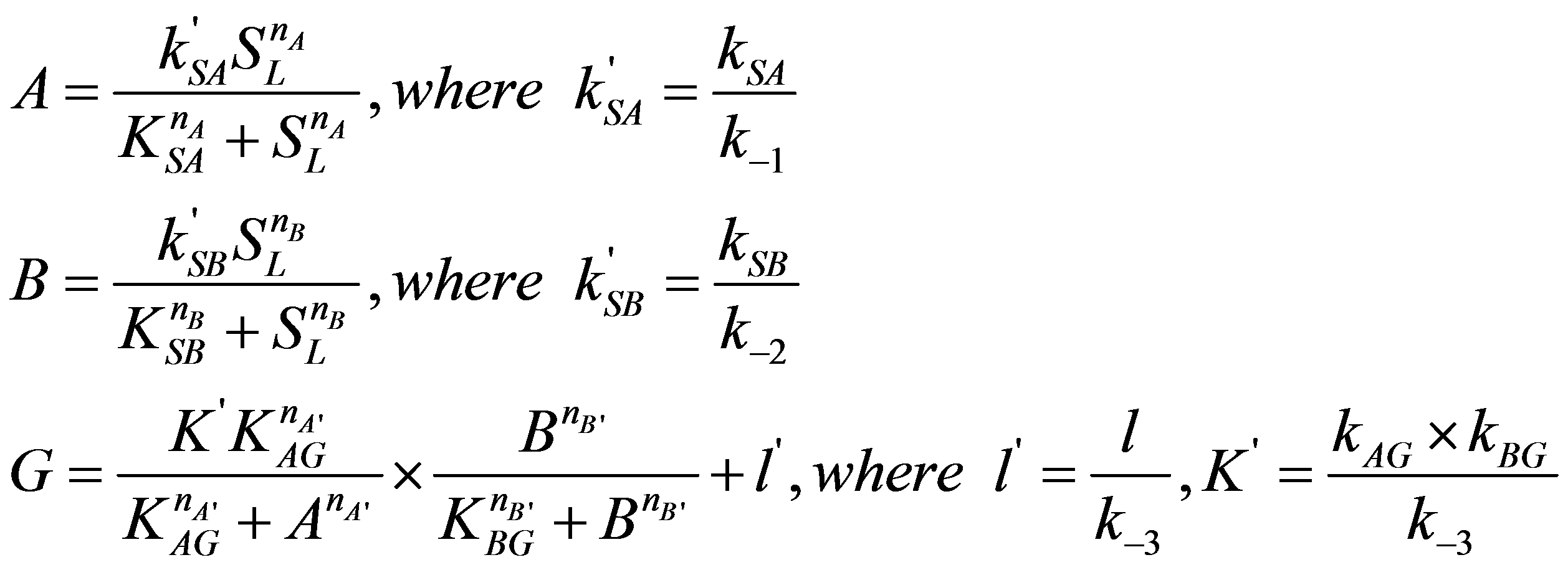Symbol usages are identical to that in ODE analysis of network topologies.

We chose one set of parameters meeting our needs (peak fold is greater than 32, peak width is less than 4 and greater than 0.5 ) as our initial parameter set. We then enlarged each parameter ten times and observed the difference between pre-change behavior and post-change behavior of the Band-pass Filter circuit. We used log(f’/f) and log(p/p’) to characterize the change in band-pass performance, where f and f’ denote the fold change before and after parameter perturbation, p and p’ denote the peak width before and after parameter perturbation. A log(f'/f) greater than zero indicates that the induction fold has increased after the parameter perturbation, meaning the perturbation is conducive to Band-pass Filter's performance. A log(p/p') greater than zero indicates that the peak width has reduced after the parameter perturbation, also meaning the perturbation is desirable.

The quantitative results of parameter sensitivity analysis are shown in Figure 5 and the qualitative results are summarized in Table 1. To further help understanding we drew a series of pictures to show the how the network behaves as some of the parameters change (Fig. 6).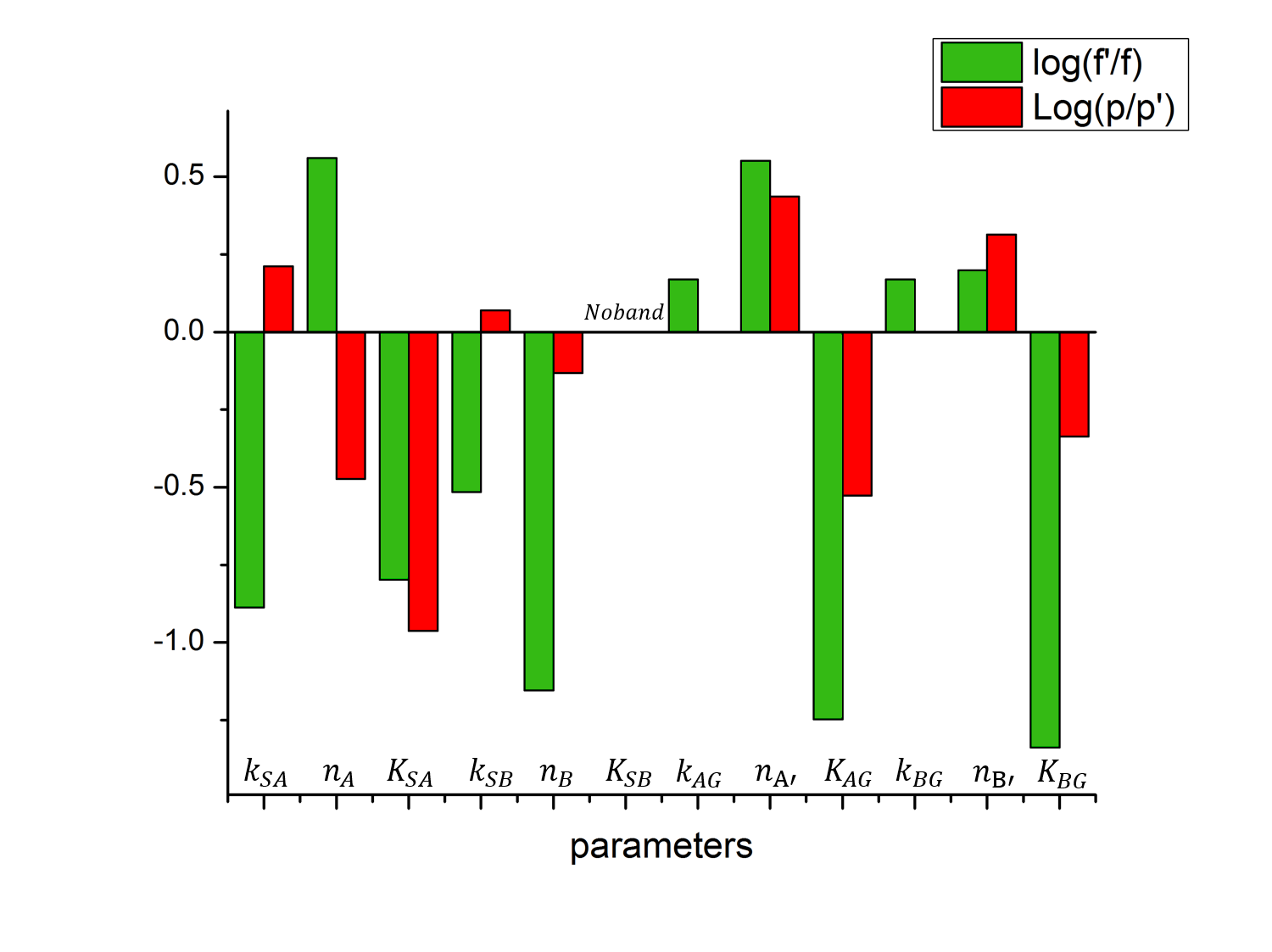Figure. 5. Histogram demonstrating performance change of Band-pass Filter circuit after parameter perturbation. Value of log(f'/f) and log(p/p') are plotted for perturbation of each parameter. f and f’ denote the fold change before and after parameter perturbation, p and p’ denote the peak width before and after parameter perturbation. An upward-pointing column indicates the perturbation is conducive to our design, and a downward-pointed column indicates the perturbation is detrimental to our design.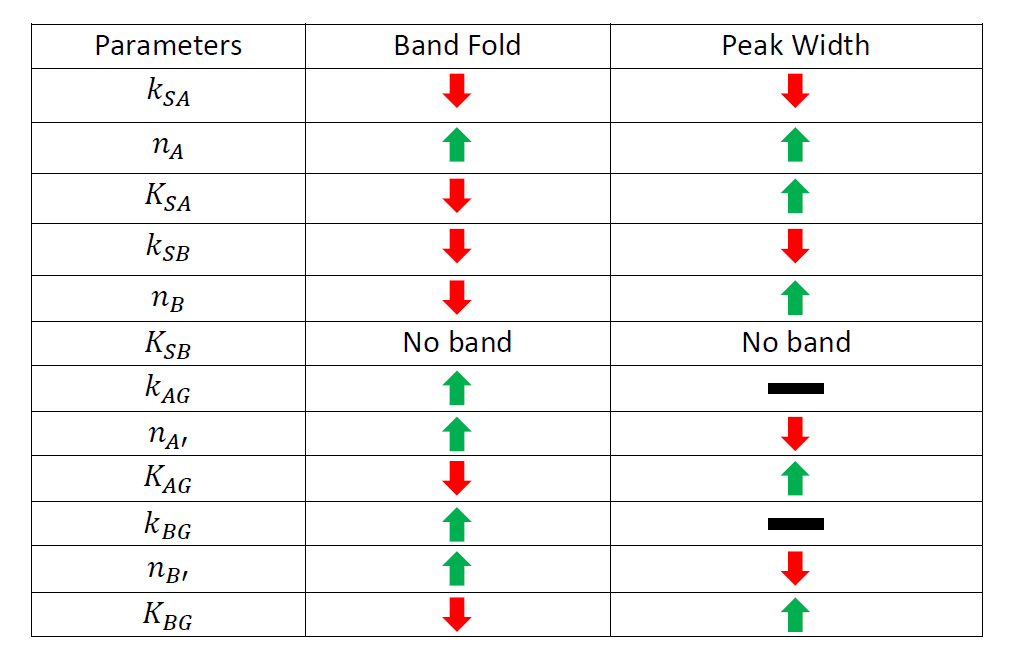Table. 1. The changing tendency of induction fold and peak width when each parameter is enlarged ten times. Green arrow indicates that the value rises as the parameter is enlarged. Red arrow indicates the reverse. Black line indicates that the value keeps constant as the parameter changes.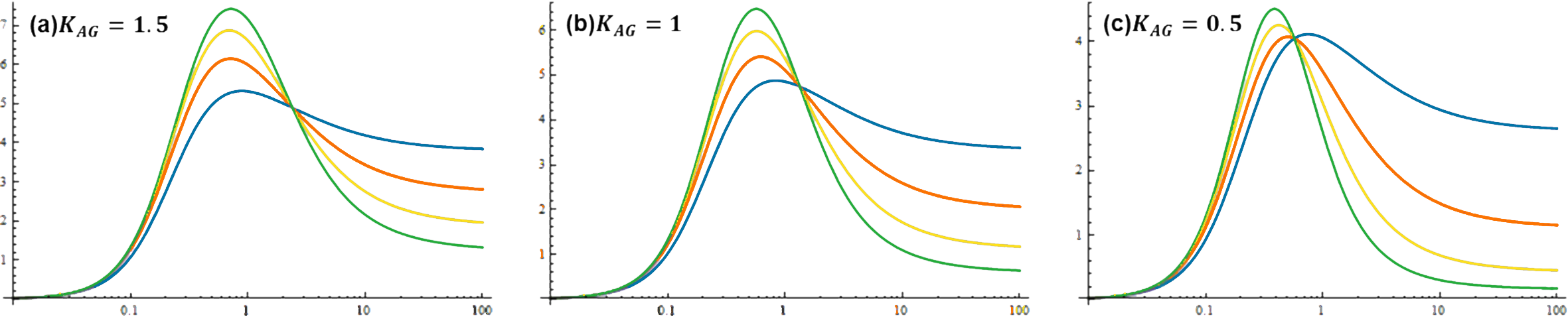Figure. 6. Dose-response curves of Band-pass Filter under different parameter sets. nA’ denotes the hill coefficient of repressor A. KAG denotes the transition concentration for repressor A. KAG value is 1.5 in a, 1.0 in b and 0.5 in c. nA' value is 2 for green lines, 1.5 for yellow lines, 1 for red lines and 0.5 for blue lines.It can be deduced from the pictures above that a small KAG and a large nA’ is conducive to the performance of Band-pass Filter.

As can be concluded from Figure 5 and Table 1, parameters are sensitive to enlargement in four different ways. Some parameters, such as kSA, nB, nA', KAG, nB' and KBG, once enlarged, will enhance or deteriorate the performance of induction ration and band width at the same time; another group of parameters, such as kSA, nA and kSB, once enlarged, will enhance one important feature while deteriorating the other; a third group of parameters, such as kAG and kBG, have little effect on one of the two important features; a final group of parameter, consisting of KSB only, will totally eliminate the band when enlarged.

In order to efficiently tune our Band-pass Filter, we decided to focus on the first group of parameters because increasing or decreasing the value of such parameters will improve the induction ratio and peak width at the same time. And since in real applications we need to change the sensor (input node) from one aromatics-responsive transcription factor to another, we didn't wish to set too tight a restriction on sensor-related parameters such as kSA and nB. So we limited the tunable parameters to nA', nB', KAG and KBG. As can be easily observed from Figure 4, for a outstanding Band-pass Filter circuit, higher values for nA' and nA' as well as lower values for KAG and KBG are desirable.

Based on all the analysis we have done above, we chose the activator ϕR73δ from phage ϕR73 as node B for its small dissociation constant (KBG) and repressor cI form phage λ as node A for its large hill coefficient (nA') to construct our final Band-pass Filter circuit (Fig. 7 and Fig.8).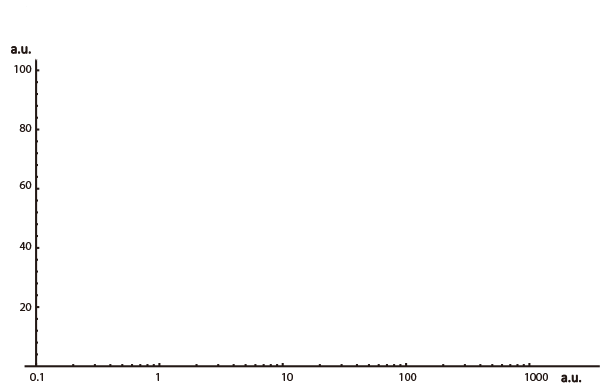Your browser does not support the HTML5 canvas tag.# Kphi=# ncI=Figure 7. An interactive image for intuitive understanding of two important parameters' function. As demonstrated in the main text, for the regulatory proteins in the band-pass filter circuit, dissociation constants need to be small and Hill coefficients need to be large in order to have a better overall performance, and we chose φR73δ and cI proteins based on such criteria. Here we set KφR73δ and ncI to be adjustable, and readers may adjust these two parameters to see the effect and establish an intuitive understanding of the two parameters' function. Have fun!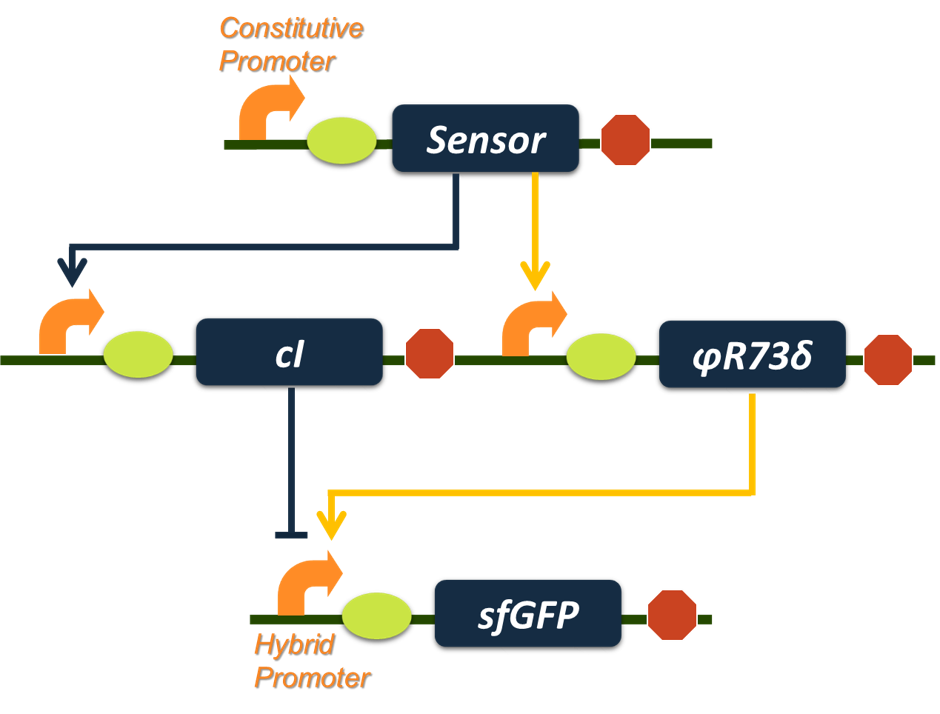Figure 8.The final construct of our Band-pass Filter. The aromatic sensor (input node) will activate transcription of φR73δ and cI gene. The φR73δ will activate transcription of sfGFP reporter gene while cI represses transcription of the reporter gene, creating an incoherent loop. With proper parameter sets, such a genetic circuit will serve the function as a Band-pass Filter.

Reference:
 SOHKA, Takayuki, et al. An externally tunable bacterial band-pass filter.Proceedings of the National Academy of Sciences, 2009, 106.25: 10135-10140.
 MA, Wenzhe, et al. Defining network topologies that can achieve biochemical adaptation. Cell, 2009, 138.4: 760-773.
 BASU, Subhayu, et al. A synthetic multicellular system for programmed pattern formation. Nature, 2005, 434.7037: 1130-1134.